Question

Calculate the standard enthalpy of reaction for the following reaction using the appropriate standard enthalpies of formation
ΔH°f [SiCl4 (g)] = -657.0 kJ/mol

SiO2 (s) + 4 HCl (g) → SiCl4 (g) + 2 H2O (g)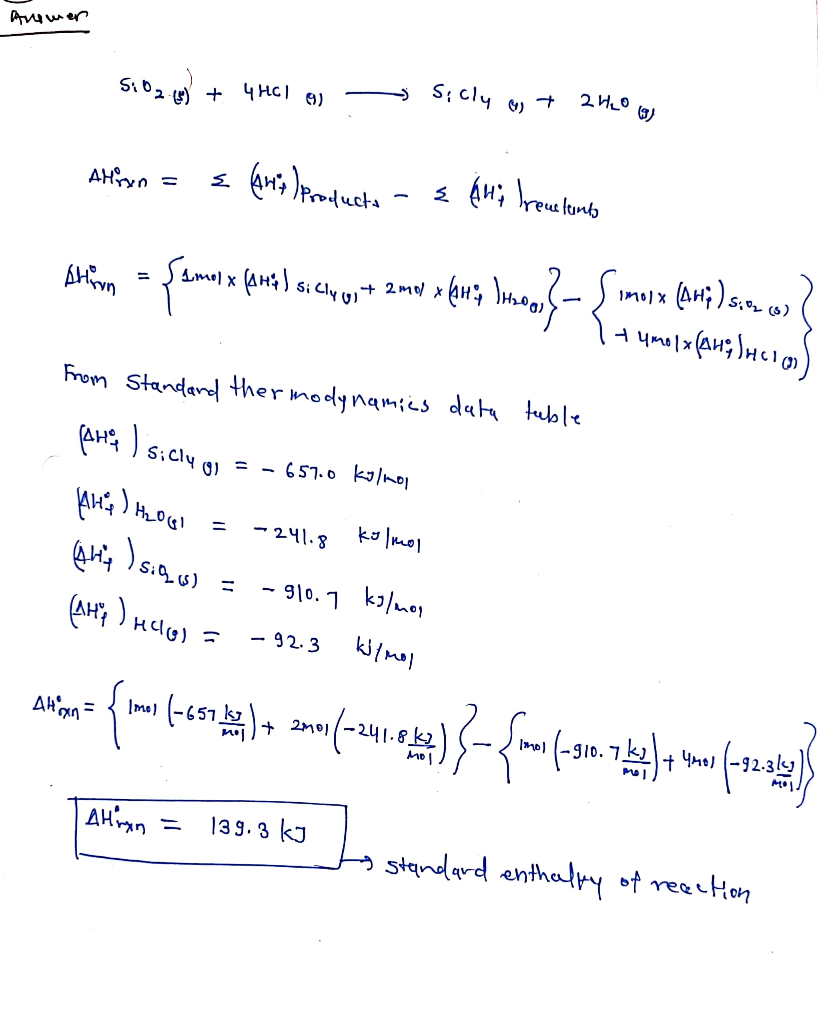#### Earn Coins

Coins can be redeemed for fabulous gifts.

Similar Homework Help Questions
• ### 12) Use the following data to calculate the standard heat (enthalpy) of formation, ???, of manganese(IV)...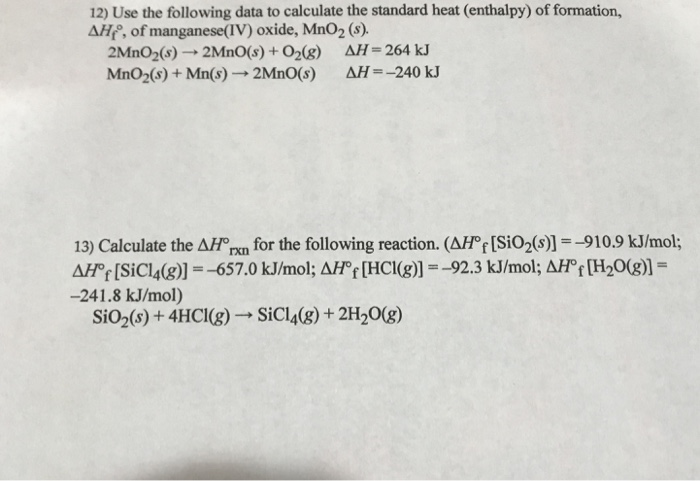12) Use the following data to calculate the standard heat (enthalpy) of formation, ???, of manganese(IV) oxide, MnO2 (s). 2MnO2(s)--2MnO(s) + O2(g) MnO2(s) + Mn(s)- 2Mn0(s) ??-264 kJ ?? -240 kJ 13) Calculate the ??0nn for the following reaction. (Arf [SiO2(s)--910.9 kJ/mol; APf [SiCl4(g)]--657.0 kJ/mol; ??'f [HCI(g)1--92.3 kJ/mol; ? Hof [H2O(g),- -241.8 kJ/mol) SiO2(s) + 4HCI(g)-SiCI(g) + 2H2O(g)

• ### help asap 3. Calculate the standard enthalpy change, Hº, for the following reaction using standard enthalpies of for...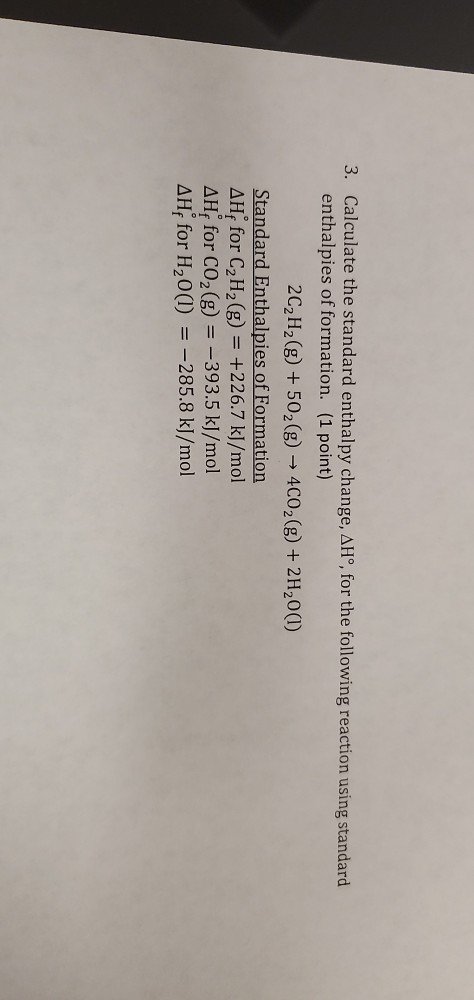help asap 3. Calculate the standard enthalpy change, Hº, for the following reaction using standard enthalpies of formation. (1 point) 2C2H2(g) + 502(g) → 4CO2(g) + 2H20(1) Standard Enthalpies of Formation AH for C2H2(g) = +226.7 kJ/mol AHfor CO2(g) = -393.5 kJ/mol AH for H2O(l) = -285.8 kJ/mol

• ### Calculate ΔH∘rxn for this reaction using standard enthalpies of formation. (The standard enthalpy of formation of...

Calculate ΔH∘rxn for this reaction using standard enthalpies of formation. (The standard enthalpy of formation of gaseous propane is -103.9 kJ/mol

• ### A scientist measures the standard enthalpy change for the following reaction to be -2847.0 kJ: 2C2H6(g)...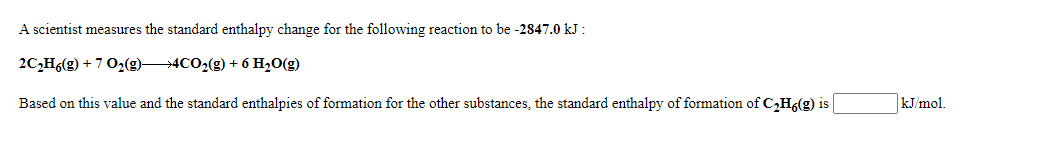A scientist measures the standard enthalpy change for the following reaction to be -2847.0 kJ: 2C2H6(g) + 7 02(g) —4CO2(g) + 6H2O(g) Based on this value and the standard enthalpies of formation for the other substances, the standard enthalpy of formation of CzH6(g) is kJ/mol. A scientist measures the standard enthalpy change for the following reaction to be -15.0 kJ : Ca(OH)2(aq) + 2 HCl(aq) +CaCl(s) + 2 H20(1) Based on this value and the standard enthalpies of formation for...

• ### A scientist measures the standard enthalpy change for the following reaction to be - 87.7 kJ : 2HBr(g) + Cl...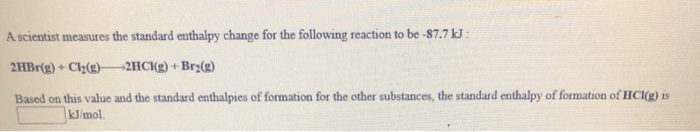A scientist measures the standard enthalpy change for the following reaction to be - 87.7 kJ : 2HBr(g) + Cl2(g)— 2HCl(g) + Bra(s) Based on this value and the standard enthalpies of formation for the other substances, the standard enthalpy of formation of HCl(g) is kJ/mol CH4() + H2O(g)_3H2(g) + CO(g) Based on this value and the standard enthalpies of formation for the other substances, the standard enthalpy of formation of H2O() is kJ/mol Given the standard enthalpy changes for...

• ### Part A Calculate the enthalpy of the reaction 2NO(g)+O2(g)→2NO2(g) given the following reactions and enthalpies of fo...

Part A Calculate the enthalpy of the reaction 2NO(g)+O2(g)→2NO2(g) given the following reactions and enthalpies of formation: 12N2(g)+O2(g)→NO2(g),   ΔH∘A=33.2 kJ 12N2(g)+12O2(g)→NO(g),  ΔH∘B=90.2 kJ Express your answer with the appropriate units. Part B Calculate the enthalpy of the reaction 4B(s)+3O2(g)→2B2O3(s) given the following pertinent information: B2O3(s)+3H2O(g)→3O2(g)+B2H6(g),    ΔH∘A=+2035 kJ 2B(s)+3H2(g)→B2H6(g),                            ΔH∘B=+36 kJ H2(g)+12O2(g)→H2O(l),                ΔH∘C=−285 kJ H2O(l)→H2O(g),                                          ΔH∘D=+44 kJ Express your answer with the appropriate units.

• ### Using standard heats of formation calculate the standard enthalpy change for the following reaction. CO(g) +...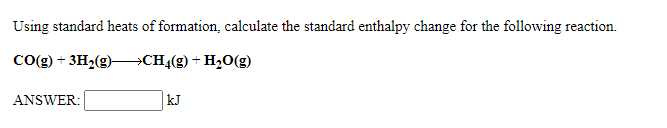Using standard heats of formation calculate the standard enthalpy change for the following reaction. CO(g) + 3H2(9)+CH_(g) + H2O(g) ANSWER: Using standard heats of formation, calculate the standard enthalpy change for the following reaction. 2502(g) + O2(g)—>2503(9) ANSWER: A scientist measures the standard enthalpy change for the following reaction to be -2847.0 kJ : 2CH (9) + 7 02(9)—4C02(g) + 6H2O(g) Based on this value and the standard enthalpies of formation for the other substances, the standard enthalpy of formation...

• ### 4. Use a standard enthalpies of formation values below to determine the change in enthalpy for...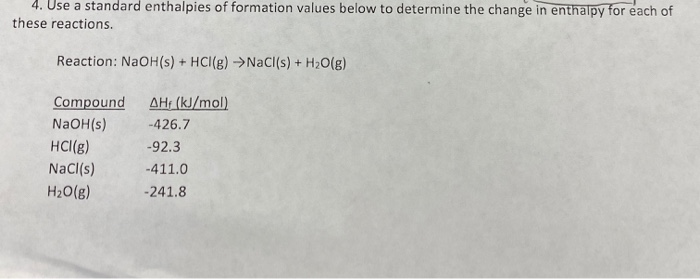4. Use a standard enthalpies of formation values below to determine the change in enthalpy for each of these reactions. Reaction: NaOH(s) + HCl(g) → NaCl(s) + H2O(g) Compound NaOH(s) HCl(g) NaCl(s) H2O(g) AHF (kJ/mol) -426.7 -92.3 -411.0 -241.8

• ### A scientist measures the standard enthalpy change for the following reaction to be -56.2 kJ :...A scientist measures the standard enthalpy change for the following reaction to be -56.2 kJ : Ca(OH)2(aq) + 2 HCl(aq)CaCl2(s) + 2 H2O(l) Based on this value and the standard enthalpies of formation for the other substances, the standard enthalpy of formation of CaCl2(s) is   kJ/mol. Answer in kJ/mol